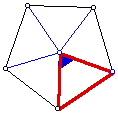# Vertex Angles in a Regular PolygonFind the measure of the vertex (blue) angle of the red isosceles triangle that can be used to rotate the triangle about its vertex to create a regular pentagon. What should the measure of the vertex angle be if you want to create a square, a regular hexagon, or a regular n-sided polygon?

Will any measure for this vertex angle in the isosceles triangle create a regular polygon? Explain why or why not.